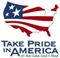Data Series 412

U.S. GEOLOGICAL SURVEY
Data Series 412

Table 1. Regression equations for the 7Q2 low-flow statistic for unregulated streams in regions 1–8 in Idaho.

[7Q2 regression equation: A, drainage area, in square miles; P, mean annual precipitation, in inches; E, mean elevation, in feet. Abbreviation: 7Q2, 7-day, 2-year low flow]

Region 7Q2 regression equation Standard error of prediction
log10 Percent
1 7Q2 = 0.300A0.879 0.271 +86.7 to -46.4
12 7Q2 = 0.000153A1.04P1.92 .253 +78.9 to -44.1
3 7Q2 = 0.0214A0.971 .277 +236 to -70.2
14 7Q2 = 0.0000215A1.04P2.41 .341 +119 to -54.4
5 7Q2 = 0.334A0.963 .249 +77.3 to -43.6
16 7Q2 = 0.000133A1.05P2.10 .243 +75.0 to -42.9
7 7Q2 = 0.0000115A0.837(E/1000)4.658 .577 +277 to -73.5
8 7Q2 = 0.00181A0.981P1.515 .331 +116 to -53.4

1 Denotes original Hortness (2006) equation.U.S. Department of the Interior | U.S. Geological Survey